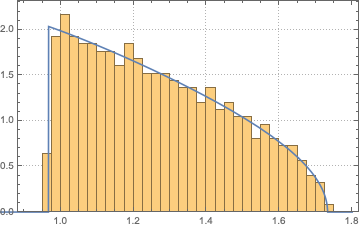# Limit of normalized sum of Dirac measures at first $\lfloor p/2\rfloor$ eigenvalues of the sample covariance matrix, with Marcenko-Pastur assumptions?

Let $$\lfloor{*}\rfloor$$ denotes the nearest integer $$\le *$$. I'm asking myself the question what's the limit of the part of the empirical spectral distribution corresponding to the first $$\lfloor{p/2}\rfloor$$ eigenvalues of the sample covariance matrix?

To be more precise, assume that $$X=[x_1,...x_n]=[x_{ij}]_{1\le i,j \le n}$$ is a $$p\times n$$ random matrix, where $$x_i$$'s are individual iid random sample, so that the entries of $$X$$, namely $$x_{ij}$$, are iid random variable with mean $$0$$, variance $$1$$ and bounded fourth moments.

Next consider: the random measure $$Head_{n,p}:=\frac{1}{\lfloor{p/2}\rfloor}\sum_{i=1}^{\lfloor{p/2}\rfloor}\delta_{\lambda_i}$$, where $$\lambda_1 \ge \lambda_2 \ge \lambda_{\lfloor{p/2}\rfloor}\ge \ldots \ge \lambda_p$$ are the eigenvalues of $$\frac{1}{p}XX^{T}$$.

Then the question is: what's the limit

$$\lim_{n,p \to \infty, p/n \to c \in (0, \infty)} Head_{n,p}?$$

Intuitively, I'd first guess that it should be the Marcenko Pastur distribution with the $$c$$ replaced by $$c/2$$, as $$\frac{\lfloor{p/2}\rfloor}{n}\to \frac{c}{2}$$ as $$\frac{p}{n}\to c$$, and that one can construct a random matrix $$Y \in \mathbb{R}^{ \lfloor{p/2}\rfloor\times n}$$ so that all the $$\lfloor{p/2}\rfloor$$ eigenvalues of $$YY^{T}$$ correspond to the first $$\lfloor{p/2}\rfloor$$ eigenvalues of $$X$$. But then the question would be what can we say about the limit of the following random measure that's a sum of Dirac measures the last $$\lfloor{p/2}\rfloor$$ eigenvalues? Mathematically that is: what's the limit of

$$Tail_{n,p}:=\frac{1}{\lfloor{p/2}\rfloor}\sum_{i=\lfloor{p/2}\rfloor}^{p}\delta_{\lambda_i}?$$

If I were correct, this should also be the Marcenko Pastur distribution with the $$c$$ replaced by $$c/2$$, but I feel it isn't.

Somewhat more generally, one can assume that $$p\to \infty$$ being a multiple of $$q$$, i.e. being of the form $$p=kq, k\to \infty$$ Let $$r, s \in \mathbb{N}$$ fixed, and then we can ask for the limit of the random measure:

$$lim_{p,n \to \infty , \frac{p}{n} \to c \in (0,\infty)}\frac{1}{sq-rq}\sum_{i=rq+1}^{sq}\delta_{\lambda_i}$$

Let me denote the Marcenko-Pastur distribution by $$\rho(\lambda)$$, so that $$\rho(\lambda)d\lambda$$ gives the probability for an eigenvalue to be in the interval $$(\lambda,\lambda+d\lambda)$$, in the limit $$p\rightarrow\infty$$ at fixed $$p/n\leq 1$$. I order the eigenvalues from large to small, $$\lambda_1\geq \lambda_2\geq \lambda_3\cdots \geq \lambda_p\geq 0$$ and define $$\Lambda_k$$ for $$1\leq k\leq p$$ by $$\int_{\Lambda_k}^\infty \rho(\lambda)d\lambda=k.$$ The marginal distribution of $$\lambda_k$$ is sharply peaked at $$\Lambda_k$$ with a width that vanishes $$\propto 1/\sqrt p$$. This is known as "spectral rigidity". As a consequence, the desired "head" distribution is simply a truncated Marcenko-Pastur, $$\rho_{\rm head}(\lambda)=\begin{cases} 2\rho(\lambda)&\text{if}\;\;\lambda<\Lambda_{p/2},\\ 0&\text{if}\;\;\lambda>\Lambda_{p/2}. \end{cases}$$ The way in which the step wise truncation is smoothed goes beyond the Marcenko-Pastur limit.

Another way to think about this, is to note that the eigenvalue distribution is self-averaging in the limit $$p\rightarrow\infty$$: you get the MP distribution from a single Wishart matrix, no need to average over many matrices; then, obviously, if you select a subset of the ordered eigenvalues of that single matrix you get the corresponding truncation of the MP distribution. This applies to any subset that contains a number of eigenvalues which scales with $$p$$, so it also applies to the more general subset in the OP --- just cut out the corresponding interval in the MP distribution.

Here is a little test for $$p=10^3$$, $$n=10^4$$, when $$\Lambda_{p/2}/n=0.966565$$: the plot compares a histogram of the 500 largest eigenvalues (rescaled by $$n$$) of a randomly generated Wishart matrix with the truncated Marcenko-Pastur. The histogram is a bit noisy (because it's drawn from a single matrix only), but it does follow the truncated MP quite closely.• The marginal distribution of $\lambda_k$ is sharply peaked at $\Lambda_k$ with a width that vanishes $\propto 1/\sqrt p$. Why? $$\rho_{\rm head}(\lambda)=\begin{cases} \rho(\lambda)&\text{if}\;\;\lambda<\Lambda_{p/2},\\ 0&\text{if}\;\;\lambda>\Lambda_{p/2}. \end{cases}$$ This doesn't make sense as $p \to \infty$. Sorry, I undestood nothing....also, math is not written the way you've written, which lacks rigor. I'm not saying your ideas are meaningless, but the way it's presented bears no rigor. Also, please cite links or name theorems. Feb 26 '20 at 21:34
• I do like and appreciate the simulation though... Feb 26 '20 at 22:25
• @Let'stalkmath -- for a derivation of the "self-averaging" property of the eigenvalue density of Wishart matrices, see Sec. 3.2 of Eigenvalue density of linear stochastic dynamical systems: A random matrix approach -- it's really a basic consequence of spectral rigidity, which applies generically to random-matrix ensembles (not only Wishart, also GOE, etc.). The answer to your query as a truncation of the MP distribution then follows directly from this property that a single matrix already produces the MP eigenvalue density. Feb 27 '20 at 8:45
• This is fine except for a factor of 2 (since it needs to integrate to 1....). That is, $\rho_{head}=2\rho$ in the window you mentioned. Feb 27 '20 at 20:43
• Certainly, factor of 2 fixed, thanks. Feb 27 '20 at 21:02